Univerza na Primorskem Fakulteta za matematiko, naravoslovje in informacijske tehnologije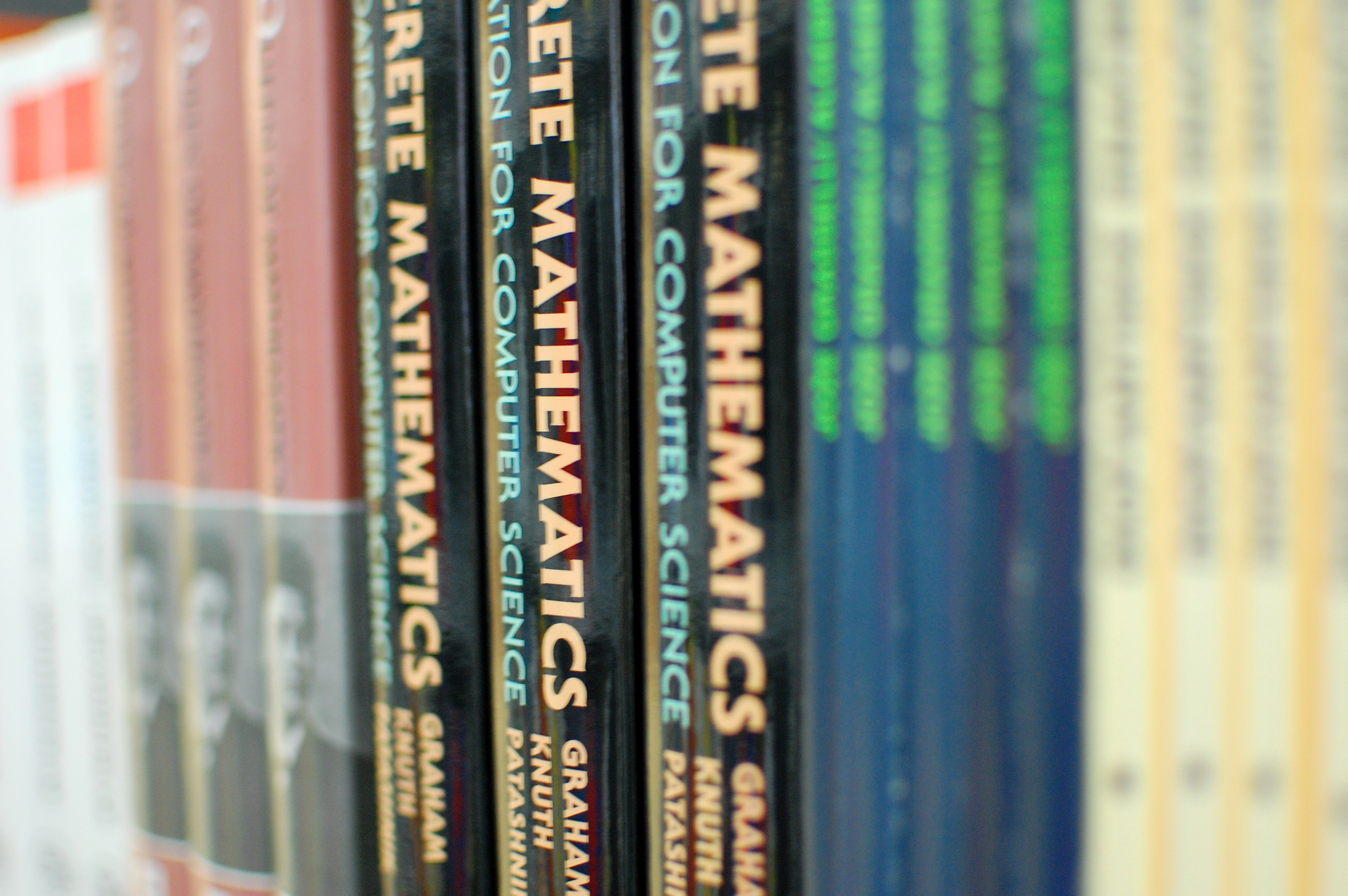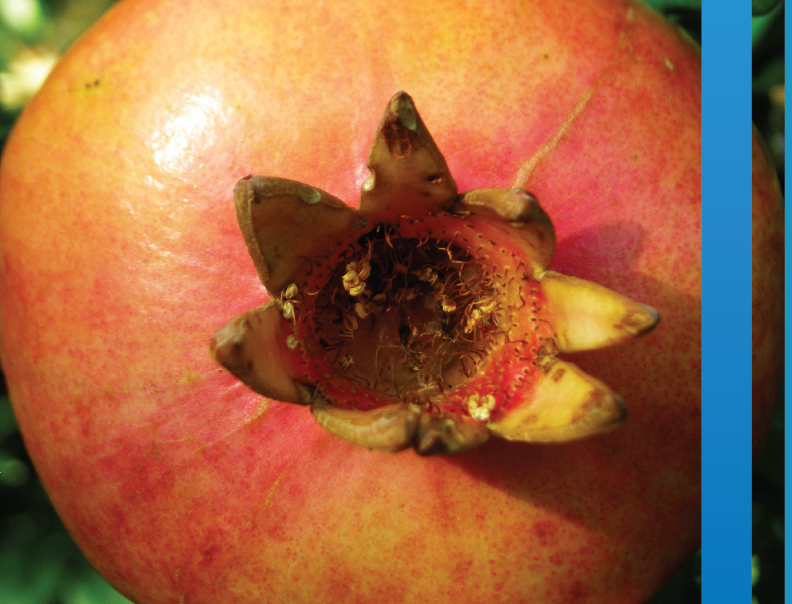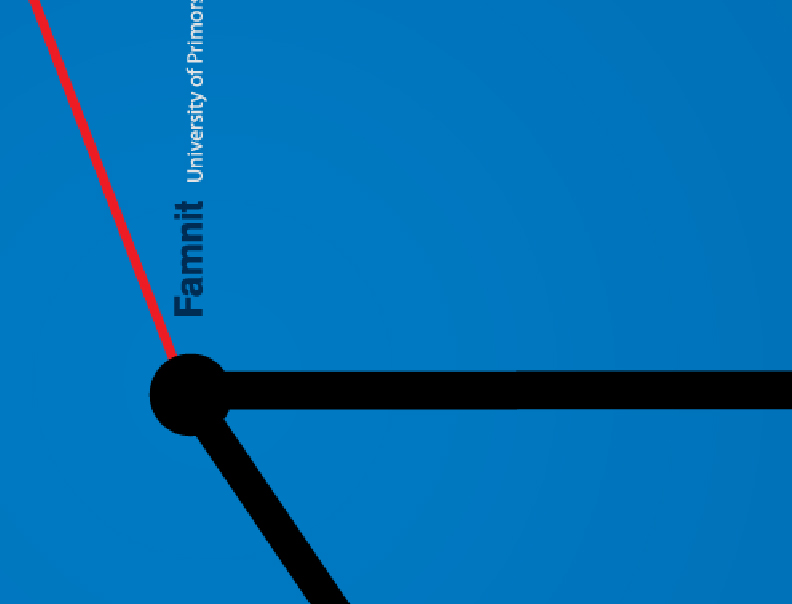SI | EN# Raziskovalni matematični seminar - Arhiv

 2021 2020 2019 2018 2017 2016 2015 2014 2013 2012 2011 2010
 1 2 3 4 5 6 7 8 9 10 11 12
Datum in ura / Date and time: 12.1.15
(10:00-11:00)
Predavalnica / Location: FAMNIT-SEMIN
Predavatelj / Lecturer: Matjaž Krnc (Faculty of Mathematics and Physics, University of Ljubljana)
Naslov / Title: Centralization of Wiener index
Vsebina / Abstract:

Freeman's centralization for a given centrality index is a measure of how central a vertex is regarding to how central all the other vertices are with respect to the given index. The well studied Wiener index (the Wiener number) of a graph G is equal to the sum of distances between all pairs of vertices of G . In the talk I will present the centralization of Wiener index, in particular, we will determine the graphs on n vertices which attain the maximum or minimum value.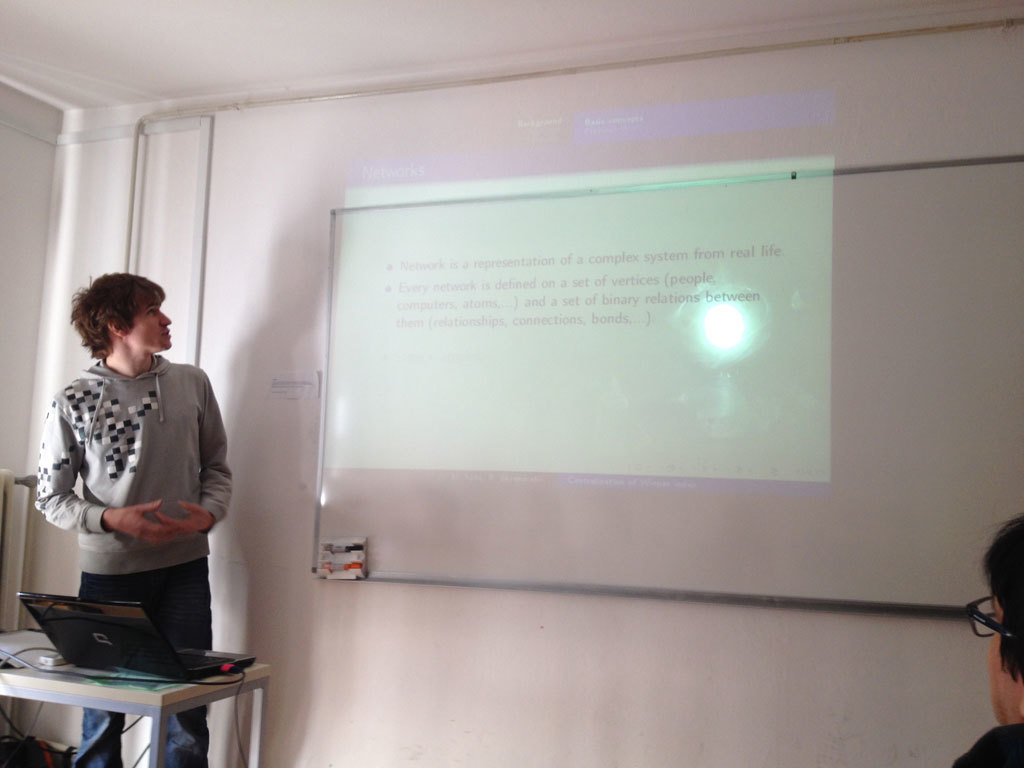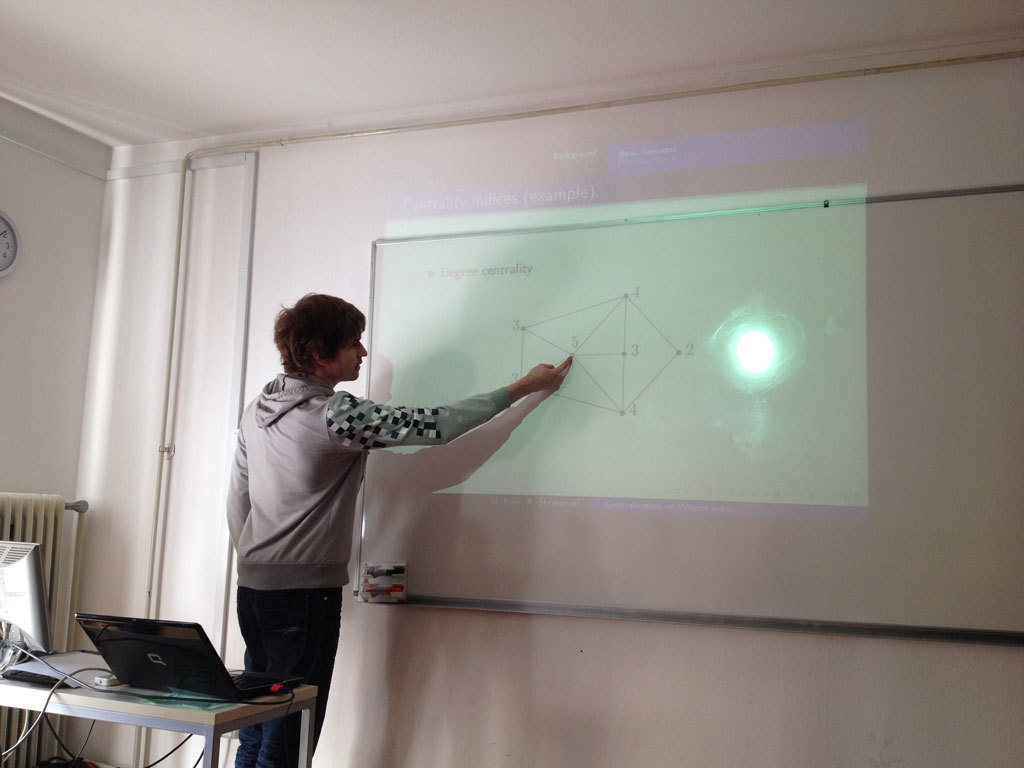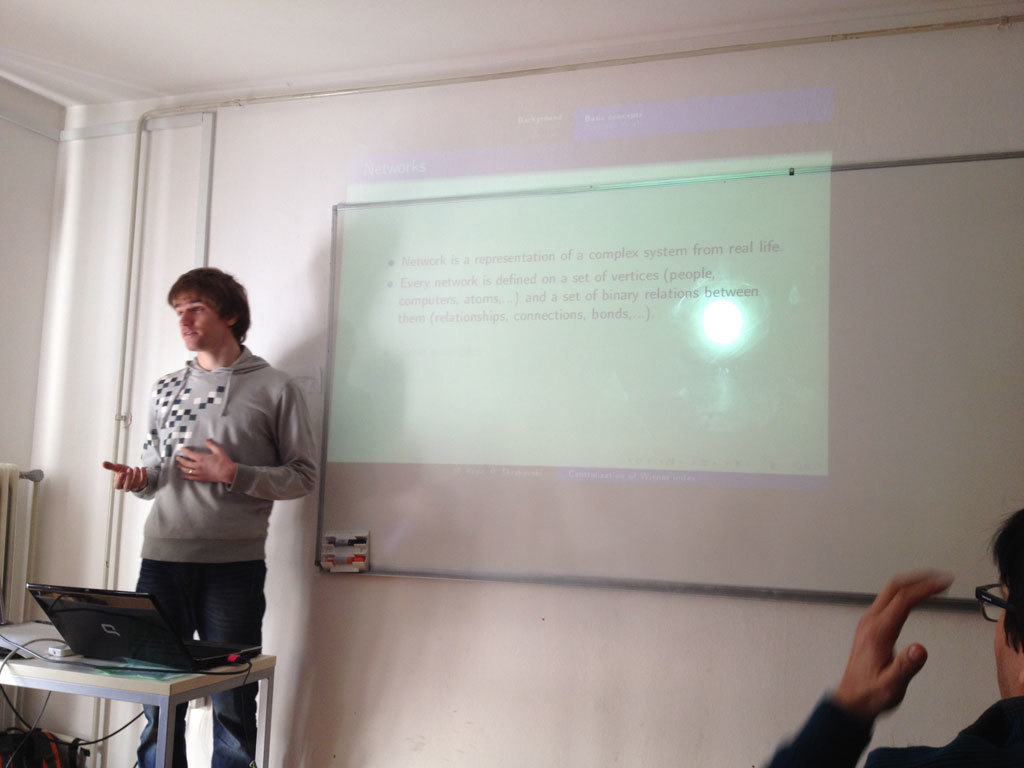Datum in ura / Date and time: 5.1.15
(10:00-11:00)
Predavalnica / Location: FAMNIT-SEMIN
Predavatelj / Lecturer: Tatiana Hartinger Romina (UP IAM)
Naslov / Title: On the structure of 1-perfectly orientable graphs
Vsebina / Abstract:

Following the terminology of Kammer and Tholey, we say that an orientation of a graph is 1-perfect if the out-neighborhood of every vertex induces a tournament, and that a graph is 1-perfectly orientable (1-p.o. for short) if it has a 1-perfect orientation. The notion of 1-p.o. graphs was introduced by Skrien (under the name B_2-graphs), where the problem of characterizing 1-p.o. graphs was posed. By definition, 1-p.o. graphs are exactly the graphs that admit an orientation that is an out-tournament. (A simple arc reversal argument shows that that 1-p.o. graphs are exactly the graphs that admit an orientation that is an in-tournament. Such orientations were called fraternal orientations in several papers.)

1-p.o. graphs form a common generalization of chordal graphs and circular arc graphs. While they can be recognized in polynomial time via a reduction to 2-SAT, little is known about their structure. We prove several results related to the structure of 1-p.o. graphs. First, we give a characterization of 1-p.o. graphs in terms of edge clique covers, similar to a known characterization of squared graphs due to Mukhopadhyay. We exhibit several examples of 1-p.o. and non-1-p.o. graphs. The examples of non-1-p.o. graphs include two infinite families: the complements of even cycles of length at least 6, and the complements of odd cycles augmented by a component consisting of a single edge. We identify several graph transformations preserving the class of 1-p.o. graphs. In particular, we show that the class of 1-p.o. graphs is closed under taking induced minors. We also study the behavior of 1-p.o. graphs under some operations that in general do not preserve the class, such as pasting along a clique and the join. The result for the join motivates the problem of characterizing the 1-p.o. co-bipartite graphs. We show that all the presented examples of non-1-p.o. graphs are minimal forbidden induced minors for the class of 1-p.o. graphs. As our main results we obtain complete characterizations of 1-p.o. graphs within the classes of complements of forests and of cographs.

Joint work with Martin Milanič.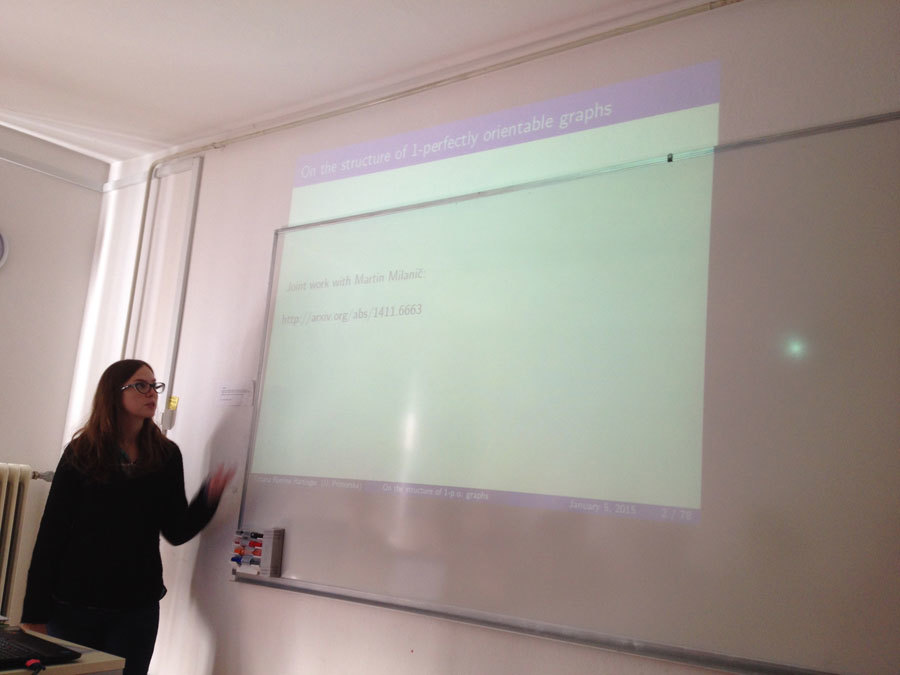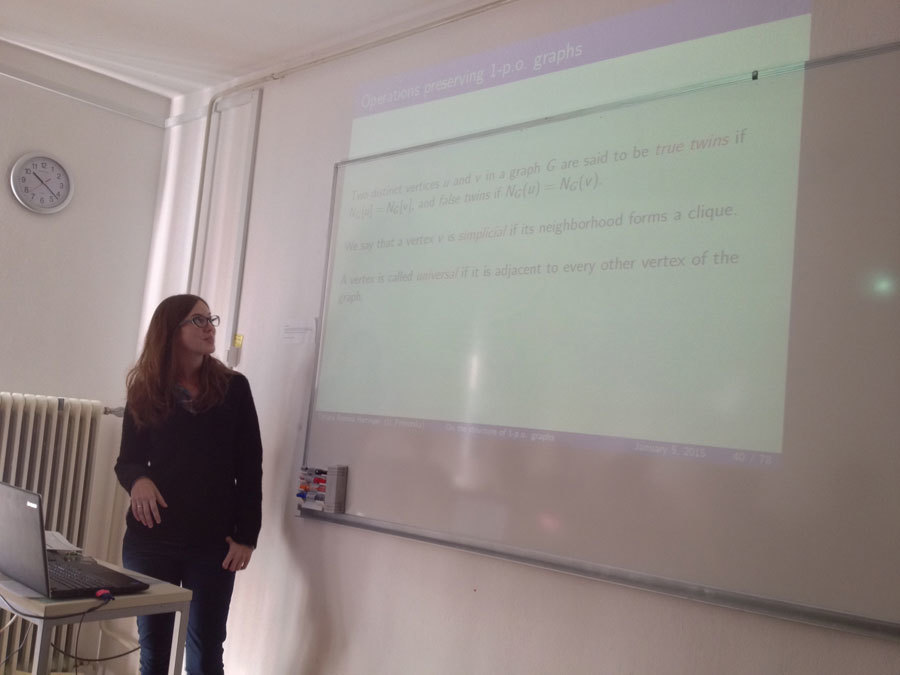http://arxiv.org/abs/1411.6663